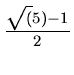Next:4.9.3 Comparison of Skip Lists and Splay TreesUp:4.9 Programming AssignmentsPrevious:4.9.1 Huffman Coding

## 4.9.2 Comparison of Hash Tables and Binary Search Trees

The objective of this assignment is to compare the performance of open hash tables, closed hash tables, and binary search trees in implementing search, insert, and delete operations in dynamic sets. You can initially implement using C but the intent is to use C++ and object-oriented principles.

Generation of Keys

Assume that your keys are character strings of length 10 obtained by scanning a text file. Call these as tokens. Define a token as any string that appears between successive occurrences of a forbidden character, where the forbidden set of characters is given by:

F = {comma, period, space}

If a string has less than 10 characters, make it up into a string of exactly 10 characters by including an appropriate number of trailing *'s. On the other hand, if the current string has more than 10 characters, truncate it to have the first ten characters only.

From the individual character strings (from now on called as tokens), generate a positive integer (from now on called as keys) by summing up the ASCII values of the characters in the particular token. Use this integer key in the hashing functions. However, remember that the original token is a character string and this is the one to be stored in the data structure.

Methods to be Evaluated

The following four schemes are to be evaluated.

1.
Open hashing with multiplication method for hashing and unsorted lists for chaining
2.
Open hashing with multiplication method for hashing and sorted lists for chaining
3.
Closed hashing with linear probing
4.
Binary search trees
Hashing Functions

For the sake of uniformity, use the following hashing functions only. In the following, m is the hash table size (that is, the possible hash values are, 0, 1,..., m - 1), and x is an integer key.

1.
Multiplication Method
h(x) = Floor(m*Fraction(k*x))

where k, the Golden Ratio.
2.
Linear Probing
h(x, i) = (h(x, 0) + imod   m;i = 0,..., m - 1
Inputs to the Program

The possible inputs to the program are:

• m: Hash table size. Several values could be given here and the experiments are to be repeated for all values specified. This input has no significance for binary search trees.
• n: The initial number of insertions of distinct strings to be made for setting up a data structure.
• M: This is a subset of the set {1, 2, 3, 4} indicating the set of methods to be investigated.
• I: This is a decimal number from which a ternary string is to be generated (namely the radix-3 representation of I). In this representation, assume that a 0 represents the search operation, a 1 represents the insert operation, and a 2 represents the delete operation.
• A text file, from which the tokens are to be picked up for setting up and experimenting with the data structure
What should the Program Do?
1.
For each element of M and for each value of m, do the following.
2.
Scan the given text file and as you scan, insert the first n distinct strings scanned into an initially empty data structure.
3.
Now scan the rest of the text file token by token, searching for it or inserting it or deleting it, as dictated by the radix-3 representation of I. Note that the most significant digit is to be considered first while doing this and you proceed from left to right in the radix-3 representation. For each individual operation, keep track of the number of probes.
4.
Compute the average number of probes for a typical successful search, unsuccessful search, insert, and delete.Next:4.9.3 Comparison of Skip Lists and Splay TreesUp:4.9 Programming AssignmentsPrevious:4.9.1 Huffman Coding
eEL,CSA_Dept,IISc,Bangalore